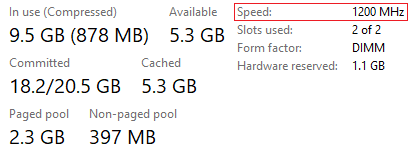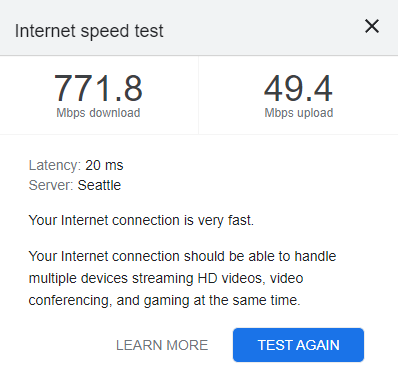Hostwinds Tutorials

Search results for:

Introduction
CPU
Memory
Disk
Network
GPU
Conversion factors
Conclusion

# What is computer-related memory and speed?

Introduction
CPU
Memory
Disk
Network
GPU
Conversion factors
Conclusion

## Introduction

The terms "memory" and "speed" are two computer-related words that can be legitimately confusing in that both terms can correctly refer to different forms of "memory" and "speed". To better understand these terms, it helps to consider the three essential components of any computer system:

• Processor
• Memory
• Disk

The processor uses its (volatile) memory and (non-volatile) disk space to do its work. And since a computer wouldn't be much fun without a connection to the internet, we can add "Network" to this list as well (with a bit more parenthesized detail):

In fact, for Windows, you can press Ctrl + Shift + Esc to see these core components in action. For example:In a nutshell, the CPU orchestrates the other components and is essentially responsible for the fact that:

• 54% of memory is being utilized.
• 3% of the disk is being utilized.
• 0% of the network is currently being utilized.
• 4% of the GPU is being utilized.

Each of these core components is discussed next.

## CPU

The CPU (central processing unit), is the "work-horse" of a computer system. Such a processor can contain multiple cores. For example, using the Windows Resource Monitor (Win + R, resmon, OK), we see that this particular processor is composed of four cores (0 through 3):Additionally, the CPU can leverage the processing power of the GPU, particularly for graphics processing, video editing, and machine learning tasks. The key metric by which CPUs are measured is clock speed, usually in GHz. A CPU running at 1 Hz can generally process one instruction per second. You can think of an "instruction" as a relatively simple calculation, such as the addition of two numbers. A CPU running at 2.9 GHz can process about 2.9 billion instructions per second, which is quite mind-boggling. For example, your average book contains about 400 pages and since each page contains about 3000 characters, a typical book contains about 1.2 million characters. If the CPU can process one character per instruction, then a 2.9 GHz CPU could process about 2416 books per second (755 pounds worth of physical books).

## Memory

Memory or RAM (random access memory) is where the CPU stores the results of its various calculations (as well as other data). This form of memory is volatile in nature meaning that when the computer is properly shut down, all data is lost by design. The key metric for memory is the amount of it, typically measured in GB. 1 GB equals 1024^3 bytes or about 1.07 billion bytes (see the conversion factor section below). Since a byte contains 8-bits (0 or 1), 1 GB of memory can contain a little over 8.5 billion bits. 8-bits can represent 2^8 = 256 characters, more than enough for your typical book. Therefore, 1 GB can store at least (1.07 billion characters) / (1.2 million characters per book) = 892 average-sized books.

Note that memory speed is also an important consideration, typically measured in MHz (millions of cycles per second).  For Windows, pressing Ctrl + Shift + Esc will start the Task Manager, which in this case shows the memory speed to be 1200 MHz:Generally, a more accurate means of measuring memory speed is via transfer rate and bandwidth:

• Transfer rate is the actual delivered speed as opposed to the frequency of the clock (clock speed). Transfer rate is typically measured in MT/s (millions of transfers per second).
• Memory bandwidth is the rate at which data can be read from and stored in memory via the CPU. Memory bandwidth is usually measured in GB/s (gigabytes per second).

In general, the faster the memory, the faster the processor can access said memory. However, the actual memory data transfer rate is generally determined by the system's CPU and motherboard configuration.

## Disk

A computer system's disk drive or drives are the non-volatile space in which the system "permanently" stores its data. Unlike RAM, when the computer system is properly shut down, this data is not (by design) lost. Compared to RAM, this form of memory is relatively slow. That said, solid-state drives (SSD) are significantly faster than traditional hard disk drives (HDD).

Like memory, the key metric for disk space is how much of it there is, usually measured in GB or TB (1 TB = 1024 GB). From the above, we know that 1 GB can contain about 892 books. Therefore, 1 TB can contain 1024 · 892 = 913,408 average-sized books using 8-bits per character. If we want to include artwork, images, and other common book items, we can use the fact that the average Kindle e-book is about 2.6 MB, so 1 TB can hold about:

``(1 book / 2.6 MB) · (1024 MB / 1 GB) · (1024 GB / 1 TB) = 403,298 books``

If we assume it takes a day to read a book, then 1 TB of disk space can provide about 1104 years of worth of continuous entertainment. Note that the multiplicative "chain" used above is explained in the conversion factor section below.

And as with memory, disk speed is also an important factor. An SSD is roughly 50 times faster than an HDD for large file transfers and roughly 200 times faster for small file (4 KB) transfers (which can make a large difference in OS speed and boot times).

## Network

The primary metric for a network is speed, usually measured in Kbps or Mbps. In fact, to quickly test your current network speed, you can use Google's Test your internet speed, an example of which follows:Note that the maximum rate of data transfer across a given path is called bandwidth and is measured in Mbps. The above test is attempting to measure the network's bandwidth.

You may have noticed that the speed test reported a 20 ms (milliseconds) latency. Latency measures how quickly you receive a response from the server. Low response times are important for real-time applications, like video calls and online gaming.

The conversion factor section below provides an example of how to calculate the time necessary to download a 2.6 MB e-book at 772 Mbps.

## GPU

The GPU or graphics processing unit, generally part of your graphics card, is primarily used for graphics rendering but the CPU can leverage the GPU to handle some of its processing, particularly around graphics processing, video editing, and machine learning. GPUs are excellent for matrix/vector operations and parallel processing. For such scenarios, you can think of the GPU as a subservient sub-component of the CPU.

GPU speed is typically measured in MHz (millions of processing cycles per second ) but unlike a CPU, other metrics such as frame rate and pixel fill rate are applicable.

## Conversion factors

We occasionally need to convert from one unit to another. For example, what's 2250 MB (megabytes) in terms of TB (terabytes)? We can reliably calculate such quantities via conversion factors.

Recall from algebra that y · (x / y) = x and x · (y / x) = y. In the first case, the leading y cancels out the y in the denominator. Similarly, in the second case, the leading x cancels out the x in the denominator. This same algebraic manipulation can be applied to units. For example, if we wanted to convert 1 cm to mm, we can treat "cm" and "mm" as algebraic quantities, as follows:

``1 cm · (10 mm / 1 cm) = 1 · (10 mm / 1) = 1 · 10 mm = 10 mm``

That is, the leading cm cancels out the cm in the denominator, leaving 10 mm. Similarly,

``17.6 cm · (10 mm / 1 cm) = 17.6 · (10 mm / 1) = 17.6 · 10 mm = 176 mm``

The quantity (10 mm / 1 cm) is known as a conversion factor, which you can "flip" as needed. For example:

``176 mm · (1 cm / 10 mm) = 176 · (1 cm / 10) = 17.6 cm``

All that's needed to create a conversion factor is a known equality. For example, if 1 hectare = 2.47105 acres, then we can convert 3.5 hectares to acres, as follows:

``3.5 hectares · (2.47105 acres / 1 hectare) = 3.5 · 2.47105 acres ≈ 8.649 acres``

Likewise, we can convert 6.649 acres back to hectares by flipping the conversion factor, as shown:

``8.649 acres · (1 hectare / 2.47105 acres) ≈ 3.5 hectares``

With that in mind, the following list may be of use with respect to memory and disk capacity conversions:

``````8 bits     = 1 byte
1024 bytes = 1 KB
1024 KB    = 1 MB
1024 MB    = 1 GB
1024 GB    = 1 TB
1024 TB    = 1 PB``````

Using the above list, we can answer the original question as follows:

``````2250 MB · (1 GB / 1024 MB) ≈ 2.1973 GB
2.1973 GB · (1 TB / 1024 GB) ≈ 0.0021 TB``````

You can also "chain" conversion factors together to perform the same calculation in one fell swoop (the only unit left standing is the one we want):

``2250 MB · (1 GB / 1024 MB) · (1 TB / 1024 GB) ≈ 0.0021 TB``

A quick web search often indicates that 1 GB = 1 billion bytes but this is not correct, as shown:

``1 GB · (1024 MB / 1 GB) · (1024 KB / 1 MB) · (1024 bytes / 1 KB) = 1,073,741,824 bytes ≅ 1.07 billion bytes ≈ 1 billion bytes``

As a final example, say we would like to estimate how long it would take to download a 2.6 MB e-book over a 772 Mbps network:

``````2.6 MB · (1024 KB / 1 MB) · (1024 bytes / KB) · (8 bits / 1 byte) = 21,810,381 bits
772 Mbps = 772 Mbit / 1 s
(772 Mbit / 1 s) · (1000 Kbit / Mbit) · (1000 bits / Kbit) = 722,000,000 bits / 1 s
21,810,381 bits · (1 s / 722,000,000 bits) ≈ 0.028 s
0.028 s · (1000 ms / 1 s) ≈ 28 ms``````

## Conclusion

A reasonable way to think about a computer system's "memory" and "speed" is in terms of the system's core components:

• Processor
• The key metric is CPU clock speed, usually measured in GHz.
• A 3.2 GHz processor executes 3.2 billion instructions per second (assuming one instruction per execution).
• The CPU can leverage the GPU for such things as graphics processing, video editing, and machine learning.
• Memory
• This is the main memory the CPU uses for its tasks.
• The key metric is memory size, usually measured in GB (1 GB = 1024^3 bytes)
• This type of memory is volatile by design, data is lost when the computer is properly shut down.
• Disk
• The key metric is disk size (or space), usually measured in GB or TB.
• This type of memory is non-volatile by design, data is generally not lost when the computer is properly shut down.
• Network.
• The key metric is network speed (data transfer rate), usually measured in Gbps (gigabits per second).
• In general, the faster the network, the faster the upload (data out) and download (data in) speeds.

Conversion factors are a handy way to convert from one unit to another, including computer system units of memory and speed.

Written by Karlito Bonnevie  /  May 20, 2022